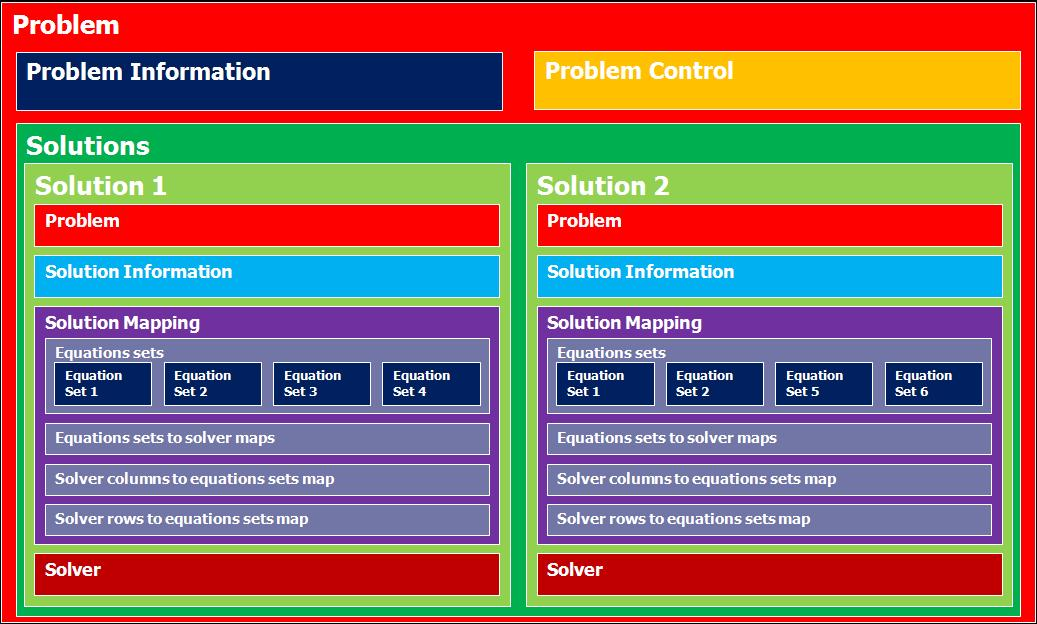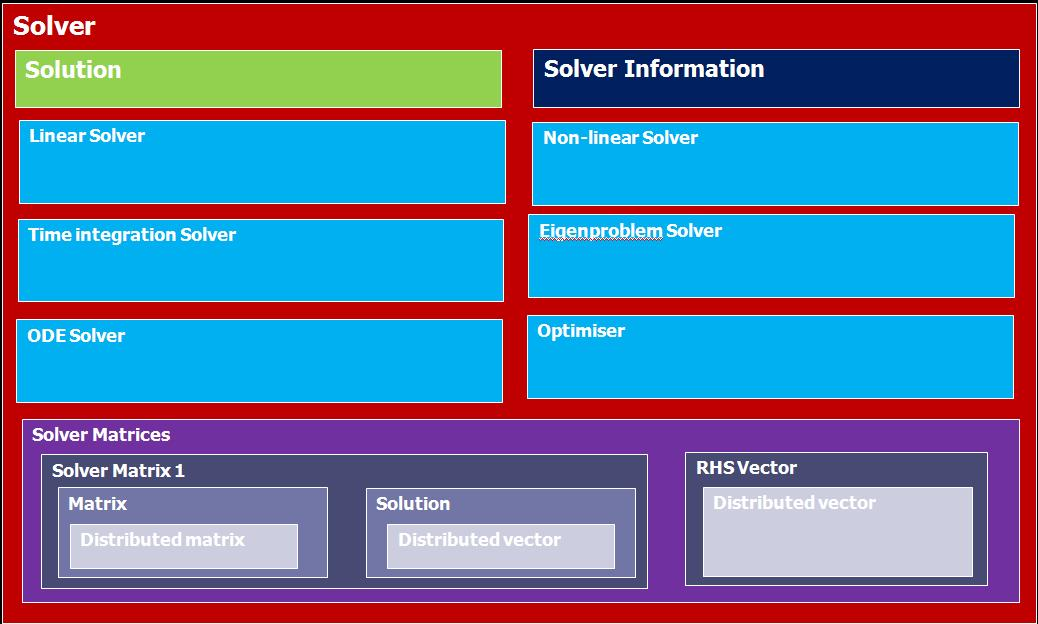OpenCMISS-Iron Internal API Documentation
Problems

A problem has a number of solutions (each with their solver) inside a problem control loop. Problem is associated with region via solution which maps to equations sets and hence links to region. Multiple problems can be in the same region, or multiple regions can work to solve one problem.

Problem has the following attributes:

• User number
• Global number
• Finished tag
• Class
• Type
• Subtype
• Control pointer
• Number of solutions
• Solutions pointer# Solutions

Solution has the following attributes:

• Solution number
• Finished tag
• Linear solution data pointer
• Nonlinear solution data pointer
• Time (non-static) solution data pointer
• Equations set to add (the next equations set to add)
• Index of added equations set(the last successfully added equations set)
• Solution mapping(which contains equations sets)
• Solver pointer

## Solvers

Solver has the following attributes:

• Solution pointer
• Finished tag
• Solve type
• Output type
• Sparsity type
• Linear solver pointer
• Non-linear solver pointer
• Time integrationn solver pointer
• Eigenproblem solver pointer
• Solver matrices# Control

Control has the following attributes:

• Problem pointer
• Finished tag
• Control type